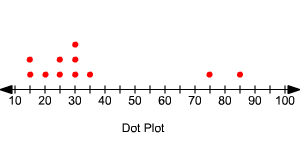# Outliers

An outlier is a value in a data set that is very different from the other values. That is, outliers are values unusually far from the middle.

In most cases, outliers have influence on mean , but not on the median , or mode . Therefore, the outliers are important in their effect on the mean.

There is no rule to identify the outliers. But some books refer to a value as an outlier if it is more than $1.5$ times the value of the interquartile range beyond the quartiles .

Also plotting the data on a number line as a dot plot will help in identifying the outliers.

Example:

Find the outliers of the data set. Also find the mean of the data set including the outliers and excluding the outliers.

$15,75,20,35,25,85,30,30,15,25,30$

First arrange the data set in order.

$15,15,20,25,25,30,30,30,35,75,85$

Plot the data on a number line as a dot plot.The values $75$ and $85$ are far off the middle. So, these two values are outliers for the given data set.

Find the mean, median and mode of the data including the outliers:

$\text{Mean}\text{\hspace{0.17em}}\text{\hspace{0.17em}}=\text{\hspace{0.17em}}\text{\hspace{0.17em}}\frac{\text{Sum}\text{\hspace{0.17em}}\text{\hspace{0.17em}}\text{of}\text{\hspace{0.17em}}\text{\hspace{0.17em}}\text{the}\text{\hspace{0.17em}}\text{\hspace{0.17em}}\text{data}\text{\hspace{0.17em}}\text{\hspace{0.17em}}\text{values}}{\text{Number}\text{\hspace{0.17em}}\text{\hspace{0.17em}}\text{of}\text{\hspace{0.17em}}\text{\hspace{0.17em}}\text{\hspace{0.17em}}\text{data}\text{\hspace{0.17em}}\text{\hspace{0.17em}}\text{values}}$

$=\frac{15+15+20+25+25+30+30+30+35+75+85}{11}$

$=35$

Find the mean of the data excluding the outliers:

$\text{Mean}\text{\hspace{0.17em}}\text{\hspace{0.17em}}=\text{\hspace{0.17em}}\text{\hspace{0.17em}}\frac{\text{Sum}\text{\hspace{0.17em}}\text{\hspace{0.17em}}\text{of}\text{\hspace{0.17em}}\text{\hspace{0.17em}}\text{the}\text{\hspace{0.17em}}\text{\hspace{0.17em}}\text{data}\text{\hspace{0.17em}}\text{\hspace{0.17em}}\text{values}}{\text{Number}\text{\hspace{0.17em}}\text{\hspace{0.17em}}\text{of}\text{\hspace{0.17em}}\text{\hspace{0.17em}}\text{\hspace{0.17em}}\text{data}\text{\hspace{0.17em}}\text{\hspace{0.17em}}\text{values}}$

$=\frac{15+15+20+25+25+30+30+30+35}{9}$

$=25$

The mean of the given data set is $35$ when outliers are included, but it is $25$ when outliers are excluded.